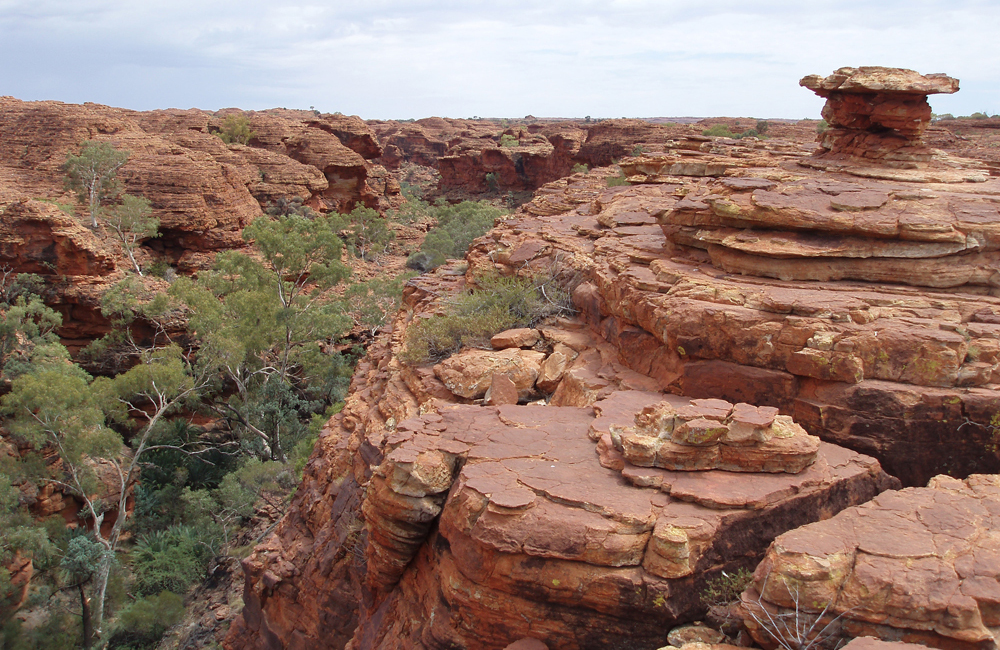Chapter 9 Statics and Torque

# 59 9.0 IntroductionFigure 1. On a short time scale, rocks like these in Australia’s Kings Canyon are static, or motionless relative to the Earth. (credit: freeaussiestock.com)

What might desks, bridges, buildings, trees, and mountains have in common—at least in the eyes of a physicist? The answer is that they are ordinarily motionless relative to the Earth. Furthermore, their acceleration is zero because they remain motionless. That means they also have something in common with a car moving at a constant velocity, because anything with a constant velocity also has an acceleration of zero. Now, the important part—Newton’s second law states that net$\boldsymbol{F=ma},$and so the net external force is zero for all stationary objects and for all objects moving at constant velocity. There are forces acting, but they are balanced. That is, they are in equilibrium.

### STATICS

Statics is the study of forces in equilibrium, a large group of situations that makes up a special case of Newton’s second law. We have already considered a few such situations; in this chapter, we cover the topic more thoroughly, including consideration of such possible effects as the rotation and deformation of an object by the forces acting on it.

How can we guarantee that a body is in equilibrium and what can we learn from systems that are in equilibrium? There are actually two conditions that must be satisfied to achieve equilibrium. These conditions are the topics of the first two sections of this chapter.